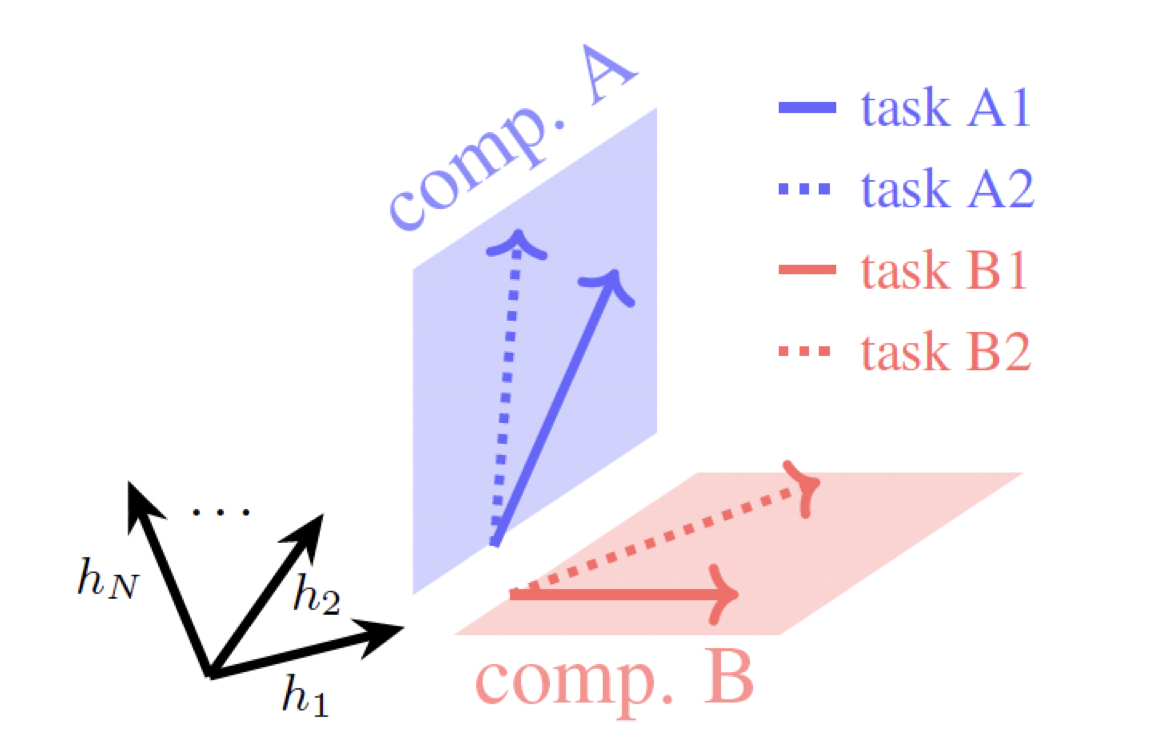# Continual learning for Recurrent Neural Networks

A few weeks ago in lab meeting we discussed ‘Organizing recurrent network dynamics by task-computation to enable continual learning’ by Duncker & Driscoll et. al 2020. This paper seeks to understand how a neural population maintains the flexibility to learn new tasks while robustly executing previously learned tasks. While biological networks learn new tasks all the time in a sequential (a.k.a. continual) fashion, continual learning in artificial neural networks has proven to be hard. These networks are known to catastrophically forget previous tasks when trained on a new task. In this work, the authors come up with a learning rule that enables a recurrent neural network to learn multiple tasks sequentially without catastrophic forgetting.

Let’s first formally define continual learning: We want to train a model on K tasks sequentially, where each task has its own set of input/output pairs represented by$\{\mathcal{D}_1, \mathcal{D}_2, ..., \mathcal{D}_K\}$. Our goal is to ensure that the network performs well per some pre-decided accuracy metric on all tasks at the end of training. The key thing to keep in mind is that, unlike simultaneous training, here we train the network on one dataset at a time, such that the network does not have access to samples from previous tasks when learning a new task.Continual learning for recurrent neural networks: This paper aims to perform continual learning using recurrent neural networks (RNNs), which are often studied as proxies for neural populations in neuroscience. The intuition behind their approach is to allow tasks that require similar computations/dynamics to use shared subspaces, while tasks that require different computations and would otherwise interfere with previously learned are encouraged to use an orthogonal subspace (see figure above).

They consider RNNs of the following form:$h_{t+1} = \phi(W^{rec} h_t + W^{in}x_t + \epsilon_t)$

where$h_t$ is the activity of hidden states at time t,$x_t$ is any external input,$\epsilon_t$ is gaussian noise and$y_t$ is the output of the network. Let’s define$W = [W^{rec}, W^{in}]$, and focus on updating this. The same ideas also hold for$W^{out}$. Typically, we update weights of a network as:$W \rightarrow W- \alpha \Delta W$ , where$\Delta W = \nabla_W \mathcal{L}$,

and$\mathcal{L}$ represents the loss function.

Project, and then project again: The authors modify this update rule such that:$\Delta W_{CL} = P_O \Delta W P_I$

Here,$P_I$ is a projection matrix that projects the weight change away from the space of all previous inputs to the network. To understand this better, let’s define the input to the hidden nodes in the network as$z_t^{k,r}=[h_t^{k,r}, x_t^{k,r}]$, at time t, trial r of task k. The projection matrix$P_I$ ensures that$\Delta W_{CL} z_t^{k,r} = 0$ for all time points within all trials of all previous tasks (this idea was introduced by Zeng et al. 2019). This preserves the network’s dynamics/outputs on any previous input, preserving its performance on all previous tasks.$P_O$, the left hand side matrix, projects the weight updates away from the output space of the network (spanned by$Wz_t^{k,r}$). The consequence of this update rule is that$W^{\top}\Delta W = 0$, which means any changes in the weights happen orthogonal to the current weight space. Tying it back to our original intuition: when the network is trained on a new task which requires any dissimilar dynamics, they are encouraged to lie on an orthogonal subspace. However, a new task can always reuse previously learned dynamics.

It works!  They test their method on a set of four neuroscience tasks (from Yang et al. 2019). The key results without going into task details are as follows:

1. Their approach is able to train a network sequentially on all the 4 tasks with better test performance across tasks as compared to existing methods and standard SGD (see figure; each color represents a new task).
2. They find that the underlying dynamics of hidden states of the network (near fixed points) for a task remains fixed even after the network is trained on newer tasks, showing that their training procedure is indeed robust!
3. They find empirical evidence that dynamics corresponding to some tasks evolve in shared subspaces (of the high-dimensional hidden states), while others use orthogonal subspaces.
4. They find organizational differences between learned dynamics through sequential training and simultaneous training: simultaneous training results in slightly better results and more efficient usage of shared structure across tasks.

Questions for future research: An important question that this approach raises is whether there is an upper limit to the number of dissimilar tasks that such a learning rule can enable the network to learn. How long can we keep projecting away from the space of outputs and inputs? Furthermore, how much does the order of tasks matter as the dynamics corresponding to newer tasks can only occupy the remaining orthogonal subspace? And finally the million dollar question: how do real neurons in the brain learn new tasks sequentially?